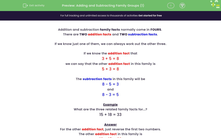# Understand Addition and Subtraction Family Groups

In this worksheet, students will show understanding that addition and subtraction are inverses and each fact has three other related facts.Key stage:  KS 1

Curriculum topic:   Number: Addition and Subtraction

Curriculum subtopic:   Understand Relationship Between Addition and Subtraction

Popular topics:   Subtraction worksheets, Addition worksheets

Difficulty level:#### Worksheet Overview

This activity is all about families.No, not that sort of family -  maths families!

Addition and subtraction family facts normally come in fours

There are two addition facts and two subtraction facts.

If we know just one of them, we can always work out the other three.

If we know the addition fact that

3 + 5 = 8

we can say that the other addition fact in this family is

5 + 3 = 8

The subtraction facts in this family will be

8 - 5 = 3

and

8 - 3 = 5

Got that?Example

What are the three related family facts for...?

15 + 18 = 33

For the other addition fact, just reverse the first two numbers.

The other addition fact in this family is

18 + 15 = 33

The subtraction facts in this family will be

33 - 15 = 18

and

33 - 18 = 15

Easy stuff, yes? Let's have a go at some questions now.

### What is EdPlace?

We're your National Curriculum aligned online education content provider helping each child succeed in English, maths and science from year 1 to GCSE. With an EdPlace account you’ll be able to track and measure progress, helping each child achieve their best. We build confidence and attainment by personalising each child’s learning at a level that suits them.

Get started